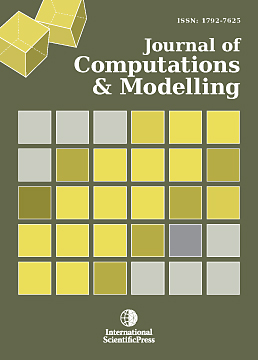# Journal of Computations & Modelling

#### A Hybrid Method for Forecasting Utilizing Genetic Algorithm With An Application to the Average Daily Number of Patients

•[ Download ]
• Abstract

High accuracy demand forecasting is essential in Supply Chain Management. In industries, how to improve forecasting accuracy such as sales, shipping is an important issue. There are many researches made on this. In this paper, a hybrid method is introduced and plural methods are compared. Focusing that the equation of exponential smoothing method(ESM) is equivalent to (1,1) order ARMA model equation, new method of estimation of smoothing constant in exponential smoothing method is proposed before by us which satisfies minimum variance of forecasting error. Generally, smoothing constant is selected arbitrarily. But in this paper, we utilize above stated theoretical solution. Firstly, we make estimation of ARMA model parameter and then estimate smoothing constants. Thus theoretical solution is derived in a simple way and it may be utilized in various fields. Furthermore, combining the trend removing method with this method, we aim to improve forecasting accuracy. An approach to this method is executed in the following method. Trend removing by the combination of linear and 2nd
order non-linear function and 3rd order non-linear function is executed to the data of the average daily number of patients for two cases (The total number of patients in hospital, Outpatients number). The weights for these functions are set 0.5 for two patterns at first and then varied by 0.01 increment for three patterns and optimal weights are searched. Genetic Algorithm is utilized to search the optimal weight for the weighting parameters of linear and non-linear function. For the comparison, monthly trend is removed after that. Theoretical solution of smoothing constant of ESM is calculated for both of the monthly trend removing data and the non monthly trend removing data. Then forecasting is executed on these data. The new method shows that it is useful for the time series that has various trend characteristics and has rather strong seasonal trend. The effectiveness of this method should be examined in various cases.ISSN: 1792-8850(Online)
1792-7625 (Print)